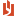## Microeconomics, Module 4: Readings for Seventh Edition

 Author Message NEASSupreme BeingGroup: Administrators Posts: 4.2K, Visits: 1.2K Microeconomics, Module 4: "Consumers in the marketplace"Readings for Seventh Edition: Landsburg, Chapter 4 (The attached PDF file has better formatting.)Updated: November 19, 2007 Module 4 applies indifference curves to practical scenarios. Changes in income and price affect the quantity demanded by consumers. We discuss wealth effects (income effects), substitution effects, and elasticities.Barro’s (macroeconomics course) also uses wealth effects and substitution effects; the concepts are the same. The regression course examines the mathematics of elasticities.For the microeconomics course, know the following about elasticities:An elasticity is the proportional change (percentage change) in the dependent variable divided by the proportional change (percentage change) in the independent variable. The dependent variable may be the quantity demanded or the quantity supplied; the independent variable may be the price, the consumer’s income, or the price of another good. If a 1% increase in price causes the quantity demanded to decrease by 2%, the price elasticity of demand is –2.We discuss four elasticities in this course: price elasticity of demand (quantity demanded / price)income elasticity of demand (quantity demanded / income)cross elasticity of demand (quantity demanded / price of another good)price elasticity of supply (quantity supplied / price) The fundamental equation is=In later chapters, we discuss the relation of elasticity and price for monopolies.If the demand curve is linear, the price elasticity of demand is high when price is high and quantity is low and low when price is low and quantity is high. If the demand curve is of the form P × Q = k, the price elasticity of demand is constant. Other postings explain why we expect the price elasticity of demand to rise or fall as the price changes.When the relation is perfectly elastic (η = 4 or –4), the graph is horizontal (flat); when the relation is completely inelastic (η = 0), the graph is vertical.Jacob: When dealing with percentage changes, we normally take logarithms. What is the relation of the logarithm to the elasticity?Rachel: If ln Q = α + β × ln P + ε, then by taking the total derivative, we getM(ln Q) = M(α + β × ln P + ε) A MQ/Q = β × MP/P A η = β.We mention this in regression analysis: if the regression is logarithmic, β is the elasticity. An example is in the time series section of the Statistics textbook.Economists use elasticities, which are not distorted by changes in the unit of measurement. It is worth knowing logarithmic regression, though Landsburg does not discuss this topic.Focus on the following items for this module:A good is normal if an increase in income increases the quantity demanded. A good is inferior if an increase in income decreases the quantity demanded. Engel curves show the relation between income and quantity demanded.Whether a good is normal or inferior depends on the consumer: poor persons buy more pasta as their incomes increaserich persons buy higher quality foods and less pasta as their incomes increase A good may be inferior when income is low but inferior when income is high.Illustration: Maccaroni and cheese, inexpensive jewelry, used cars, and cheeseburgers are normal goods for low income consumers and inferior goods for high income consumer. Yachts, lamb chops, and residential land are normal goods even for wealthy consumers.Final exam: Expect a question asking you to derive the price elasticity of demand or supply at a given point from the demand or supply curve. The demand or supply curve may be any form, though we generally use linear curves.Final exam: Expect a question asking if the price elasticity of demand is higher or lower as price or quantity increases or decreases (if the demand curve is linear).Homework assignments: The homework asks you to discuss the elasticity of insurance, to see if you have read the textbook.Landsburg shows how to derive the demand curve from the indifference curves, seeing how the consumer’s optimum changes as the price of the good changes. The analysis is complex and is never done in practice; we do not test it on the final exam.For a Giffen good, the quantity demanded increases when its price increases. A Giffen good does not contradict the law of demand, since the pure substitution effect obeys the relation: quantity demanded falls as the price rises. If the good is inferior and consumers spend most of their income on this good, it may be Giffen. Giffen goods occur in theory, but rarely (if ever) in practice. An economist named Giffen theorized that farmers ate more potatoes during a mid-19th century Irish famine when the price of potatoes rose because they could not afford more expensive foods. Many economists doubt that this happened. For consumers in the U.S. and other industrialized countries, there are no Giffen goods.Landsburg shows graphs for compensated demand curves and uncompensated demand curves. We do not test these on the final exam. Skip pages 96-96 on the compensated demand curve. AttachmentsMicro.Module4.readings.Ed7.pdf (801 views, 49.00 KB)
##### Merge Selected
Merge into selected topic...

Merge into merge target...

Merge into a specific topic ID...